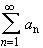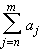#Interactive Real Analysis

Next | Previous | Glossary | Map

## 4.1. Series and Convergence

### Theorem 4.1.9: Cauchy Criteria for Series

The seriesconverges if and only if for every> 0 there is a positive integer N such that if m > n > N then || <### Proof:

Suppose that the Cauchy criterion holds. Pick any> 0. Then
| S n - S m | = || <But that means precisely that the sequence of partial sums { S N } is a Cauchy sequence, and hence convergent.

Now suppose that the sum converges. Then, by definition, the sequence of partial sums converges. In particular, that sequence must be a Cauchy sequence: given any> 0, there is positive integer N such that whenever n, m > N we have that

| S n -S m | = || <But that, in turn, means that the Cauchy criterion for series holds.Next | Previous | Glossary | Map